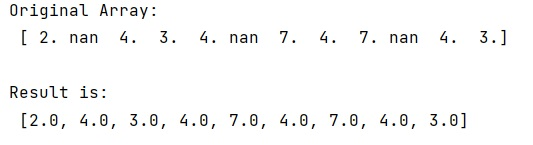# How can I remove Nan from list NumPy array?

Learn, how to remove Nan from list NumPy array? By Pranit Sharma Last updated : October 08, 2023

NumPy is an abbreviated form of Numerical Python. It is used for different types of scientific operations in python. Numpy is a vast library in python which is used for almost every kind of scientific or mathematical operation. It is itself an array which is a collection of various methods and functions for processing the arrays.

While creating a DataFrame or importing a CSV file, there could be some NaN values in the cells. NaN values mean "Not a Number" which generally means that there are some missing values in the cell.

## Problem statement

Suppose we are given an Empire array with some Nan values and we need to remove these Nan values from this NumPy array or we need to extract an array without any Nan value present in it.

## Removing Nan from list NumPy array

For this purpose, we will create a list of the elements of an array where we will select only those elements which are not nan.

Let us understand with the help of an example,

## Python program to remove Nan from list NumPy array

```# Import numpy
import numpy as np

# Creating a numpy array
arr = np.array([2,np.nan,4,3,4,np.nan,7,4,7,np.nan,4,3])

# Display original array
print("Original Array:\n",arr,"\n")

# Determining whether arr is numeric or not
res = [ x for x in arr if str(x) != 'nan']

# Display result
print("Result is:\n",res,"\n")
```

### Output

The output of the above program is: